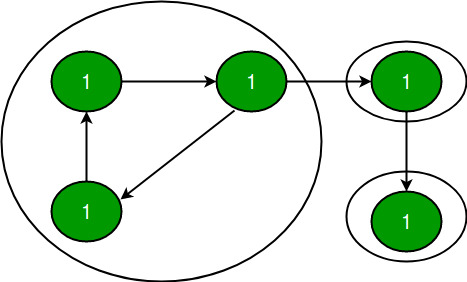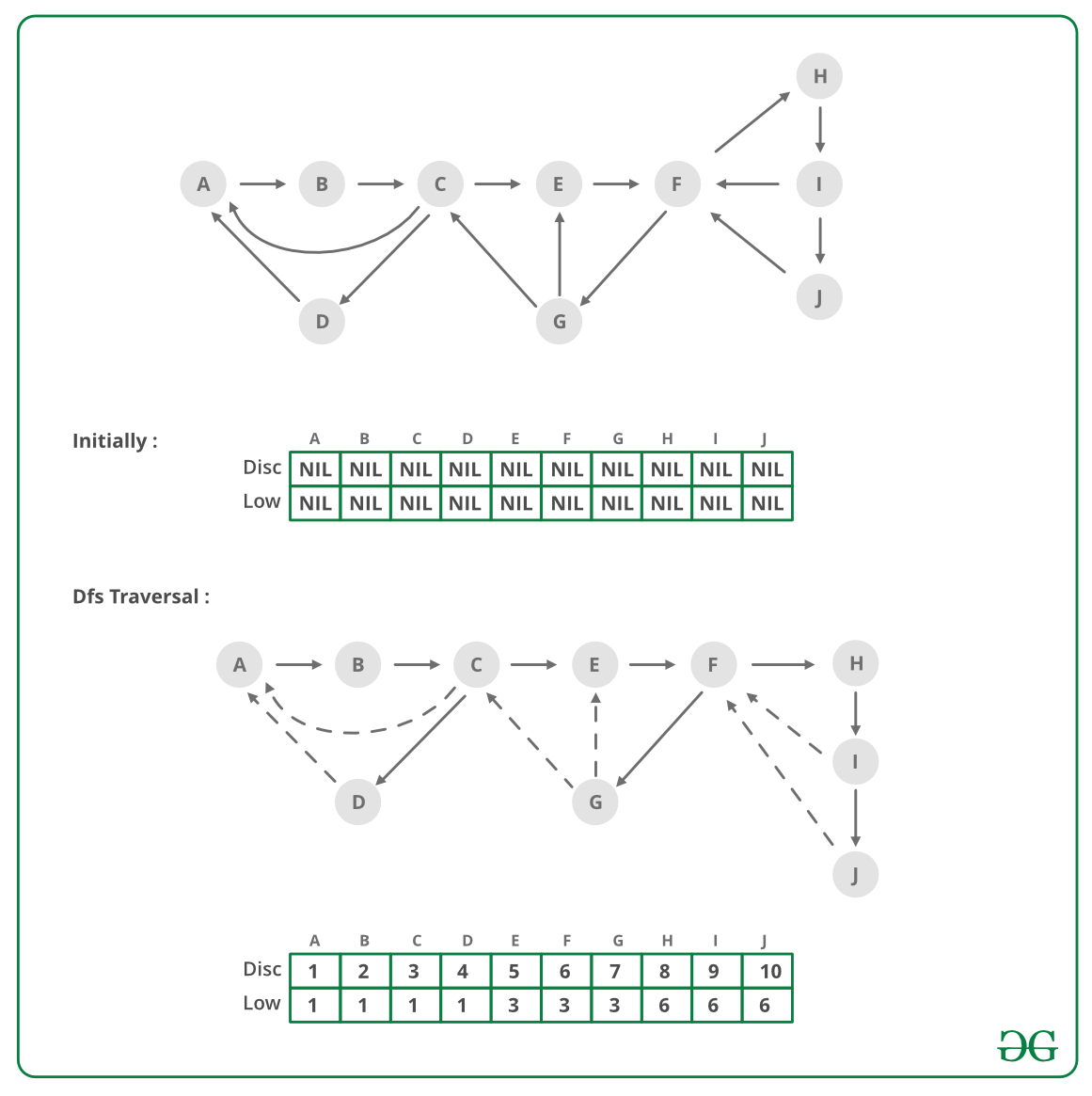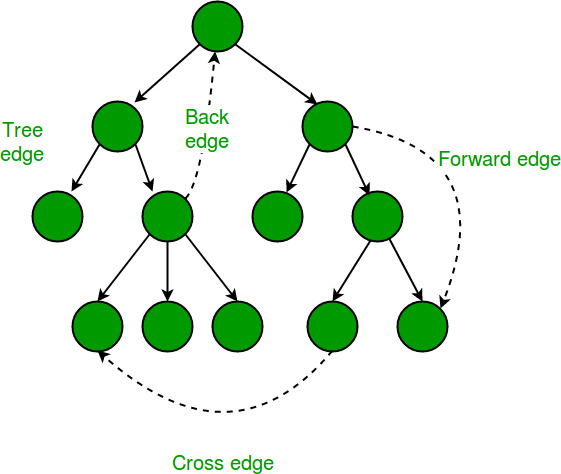Tarjan’s Algorithm to find Strongly Connected Components

• Difficulty Level : Hard
• Last Updated : 14 Jan, 2022

A directed graph is strongly connected if there is a path between all pairs of vertices. A strongly connected component (SCC) of a directed graph is a maximal strongly connected subgraph. For example, there are 3 SCCs in the following graph.We have discussed Kosaraju’s algorithm for strongly connected components. The previously discussed algorithm requires two DFS traversals of a Graph. In this post, Tarjan’s algorithm is discussed that requires only one DFS traversal.

Tarjan Algorithm is based on following facts:
1. DFS search produces a DFS tree/forest
2. Strongly Connected Components form subtrees of the DFS tree.
3. If we can find the head of such subtrees, we can print/store all the nodes in that subtree (including head) and that will be one SCC.
4. There is no back edge from one SCC to another (There can be cross edges, but cross edges will not be used while processing the graph).

To find head of a SCC, we calculate disc and low array (as done for articulation point, bridge, biconnected component). As discussed in the previous posts, low[u] indicates earliest visited vertex (the vertex with minimum discovery time) that can be reached from subtree rooted with u. A node u is head if disc[u] = low[u].

Below image is an illustration of the approach:Strongly Connected Component relates to directed graph only, but Disc and Low values relate to both directed and undirected graph, so in above pic we have taken an undirected graph.
In above Figure, we have shown a graph and its one of DFS tree (There could be different DFS trees on same graph depending on order in which edges are traversed).

In DFS tree, continuous arrows are tree edges and dashed arrows are back edges (DFS Tree Edges
Disc and Low values are showin in Figure for every node as (Disc/Low).

Disc: This is the time when a node is visited 1st time while DFS traversal. For nodes A, B, C, .., J in DFS tree, Disc values are 1, 2, 3, .., 10.
Low: In DFS tree, Tree edges take us forward, from ancestor node to one of its descendants. For example, from node C, tree edges can take us to node node G, node I etc. Back edges take us backward, from a descendant node to one of its ancestors. For example, from node G, Back edges take us to E or C. If we look at both Tree and Back edge together, then we can see that if we start traversal from one node, we may go down the tree via Tree edges and then go up via back edges. For example, from node E, we can go down to G and then go up to C. Similarly from E, we can go down to I or J and then go up to F. “Low” value of a node tells the topmost reachable ancestor (with minimum possible Disc value) via the subtree of that node. So for any node, Low value equal to its Disc value anyway (A node is ancestor of itself). Then we look into its subtree and see if there is any node which can take us to any of its ancestor. If there are multiple back edges in subtree which take us to different ancestors, then we take the one with minimum Disc value (i.e. the topmost one). If we look at node F, it has two subtrees. Subtree with node G, takes us to E and C. The other subtree takes us back to F only. Here topmost ancestor is C where F can reach and so Low value of F is 3 (The Disc value of C).

Based on above discussion, it should be clear that Low values of B, C, and D are 1 (As A is the topmost node where B, C and D can reach). In same way, Low values of E, F, G are 3 and Low values of H, I, J are 6.
For any node u, when DFS starts, Low will be set to its Disc 1st

Then later on DFS will be performed on each of its children v one by one, Low value of u can change it two case:
Case1 (Tree Edge): If node v is not visited already, then after DFS of v is complete, then minimum of low[u] and low[v] will be updated to low[u].
low[u] = min(low[u], low[v]);
Case 2 (Back Edge): When child v is already visited, then minimum of low[u] and Disc[v] will be updated to low[u].
low[u] = min(low[u], disc[v]);

In case two, can we take low[v] instead of disc[v] ?? . Answer is NO. If you can think why answer is NO, you probably understood the Low and Disc concept.Same Low and Disc values help to solve other graph problems like articulation point, bridge and biconnected component.
To track the subtree rooted at head, we can use a stack (keep pushing node while visiting). When a head node found, pop all nodes from stack till you get head out of stack.

To make sure, we don’t consider cross edges, when we reach a node which is already visited, we should process the visited node only if it is present in stack, else ignore the node.

Recommended: Please solve it on “PRACTICE” first, before moving on to the solution.

Following is implementation of Tarjan’s algorithm to print all SCCs.

Javascript



Output:

SCCs in first graph
4
3
1 2 0

SCCs in second graph
3
2
1
0

SCCs in third graph
5
3
4
6
2 1 0

SCCs in fourth graph
8 9
7
5 4 6
3 2 1 0
10

SCCs in fifth graph
4 3 2 1 0

Time Complexity: The above algorithm mainly calls DFS, DFS takes O(V+E) for a graph represented using adjacency list.

References:
http://en.wikipedia.org/wiki/Tarjan%27s_strongly_connected_components_algorithm
http://www.ics.uci.edu/~eppstein/161/960220.html#sca